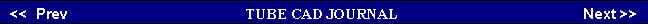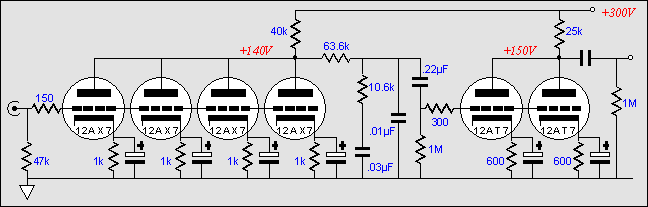The logic is simple: particularly, in a phono stage, where millivolt signals are amplified, we do not want any interaction between the triodes in a tube envelope. With all the triodes in an envelope seeing the same input signal, the interaction is minimized.     The calculation of the equalization network's values requires taking into account the output impedance of the first stage and the input resistance and capacitance of the second gain stage. Assuming a bypassed cathode resistor, the output impedance is easily derived from taking the effective rp of the input tubes in parallel and placing this value in parallel with the plate resistor's value, which is added to the 63.6k resistor's value. The input capacitance, i.e. the Miller-effect capacitance, is derived from taking the cathode-to-plate capacitance and multiplying it by the gain of the second grounded-cathode amplifier and then adding this sum to the grid-to-cathode capacitance. This capacitance adds to the .01 µF capacitor's value, which brings the equalization network into the correct alignment.      Of course, a two-gain stage, passive equalization layout is not limited to grounded-cathode amplifiers. In fact, as long as there are two gain stages bridged by a passive equalization network, just about any tube amplifier circuit can be used: the SRPP, the cascode, the grounded-grid amplifier (with some tweaking), and the common-cathode amplifier. Other circuits that could be used are the constant-current-draw amplifier, the plate follower, and the two tube feedback pair.
 Parallel Tubes    The usual disadvantage of using many tubes in parallel is the greatly increased input capacitance, but in a phono preamp this capacitance works in our favor. Phono cartridges like to be loaded by capacitance, as the capacitor's declining impedance with increasing frequency helps to damp the cartridge's high frequency resonances. And the second gain stage's increased input capacitance only helps to complete the passive equalization network's own internal need for a final shunting capacitance.    On the other hand, the usual advantage to many parallel tubes lies in the lower output impedance and lower noise figure, both being highly desirable in a phono preamp. Like many resistors held in parallel, many tubes held in parallel lower the effective resistance of the bundle. In other words, the effective rp of 6 12AX7s held in parallel is one sixth (about 10k) that of one 12AX7 (about 62k).    Furthermore, the triode's own rp gives rise to a noise similar to the noise a high resistance resistor would bring to a circuit. And like the resistor, the lower the effective rp, the lower the resistance noise. In other words, six 12AX7s held in parallel are six times lower in this type of noise than a single 12AX7. Why not use three or five triodes in parallel? In other words, why should only an even number of triodes be used? If the triode you are using comes one to the envelope, then any number of triodes can be placed in parallel. But if the triode comes two to an envelope, such as the 6DJ8, 6N1P or 12AX7 does, then an even number is better. Why?www.tubecad.com   Copyright © 2002 GlassWare   All Rights Reserved

Pg.

2